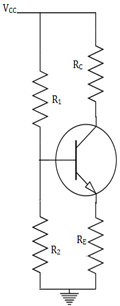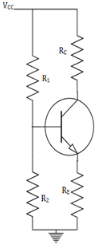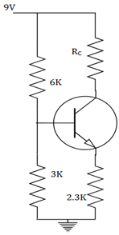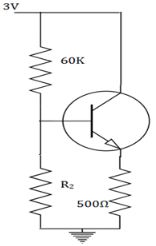Courses

# Test: Self Bias

## 10 Questions MCQ Test Electronic Devices | Test: Self Bias

Description
This mock test of Test: Self Bias for Electrical Engineering (EE) helps you for every Electrical Engineering (EE) entrance exam. This contains 10 Multiple Choice Questions for Electrical Engineering (EE) Test: Self Bias (mcq) to study with solutions a complete question bank. The solved questions answers in this Test: Self Bias quiz give you a good mix of easy questions and tough questions. Electrical Engineering (EE) students definitely take this Test: Self Bias exercise for a better result in the exam. You can find other Test: Self Bias extra questions, long questions & short questions for Electrical Engineering (EE) on EduRev as well by searching above.
QUESTION: 1

### The collector current (IC) that is obtained in a self biased transistor is_________

Solution:

The collector current is analysed by the DC analysis of a transistor. It involves the DC equivalent circuit of a transistor. The base current is first found and the collector current is obtained from the relation, IC=IBβ.

QUESTION: 2

### The collector to emitter voltage (VCE) is obtained by_________

Solution:

The collector to emitter voltage is obtained in order to find the operating point of a transistor. It is taken when there is no signal applied to the transistor. The point thus obtained lies in the cut off region when the transistor is used as a switch.

QUESTION: 3

### The thermal runway is avoided in a self bias because_________

Solution:

The self destruction of a transistor due to increase temperature is called thermal run away. It is avoided by the negative feedback produced by the emitter resistor in a self bias. The IC which is responsible for the damage is reduced by decreased output signal.

QUESTION: 4

When the temperature is increased, what happens to the collector current after a feedback is given?

Solution:

Before the feedback is applied, when the temperature is increased, the reverse saturation increases. The collector current also increases. When the feedback is applied, the drop across the emitter resistor increases with decreasing collector current and the thermal runway too.

QUESTION: 5

What is the Thevenin’s voltage (VTH) in a self bias shown below?Solution:

The base current cannot be obtained directly from the KVL or KCL applications. The VCC and VBE cannot come under a single equation. So, the circuit is changed with a Thevenin’s voltage (VTH) and Thevenin’s resistance.

QUESTION: 6

What is the Thevenin’s resistance (RTH) in a self bias shown below?Solution:

The base current cannot be obtained directly from the KVL or KCL applications. A potential divider network is formed by R1 and R2.The VCC and VBE cannot come under a single equation. So, the circuit is changed with a Thevenin’s resistance.

QUESTION: 7

The stability factor for a self biased transistor is_________

Solution:

The stability of the circuit is inversely proportional to the stability factor. The emitter resistor is very large when compared to the Thevenin’s resistance. When β is not that large, then S=(1+ β)( RTH+ RE)/ (1+ β)RE+ RTH.

QUESTION: 8

In the circuit, the transistor has a large β value (VBE=0.7V). Find the current through RC.Solution:

We know, IC=VTH-VBE/RE
=9*3/9=3V. IC=3-0.7/2.3=1mA.

QUESTION: 9

A silicon NPN transistor is used and it has a large value of β. Find the required value of R2when IC=1mA.​Solution:

For silicon, VBE=0.8V, VCE=0.2V. IC=VTH-VBE/RE. By pitting the values, we have VTH=1.3V. R2 can be found from, VCCR2/R1+R2. We get R2=40KΩ.

QUESTION: 10

The value of αac for all practical purposes, for commercial transistors range from_________

Solution:

For all practical purposes, αac=αdc=α and practical values in commercial transistors range from 0.9-0.99. It is the measure of the quality of a transistor. Higher is the value of α, better is the transistor in the sense that collector current approaches the emitter current.